# NCERT Solutions For Class 11 Physics Waves## myCBSEguide App

Complete Guide for CBSE Students

NCERT Solutions, NCERT Exemplars, Revison Notes, Free Videos, CBSE Papers, MCQ Tests & more.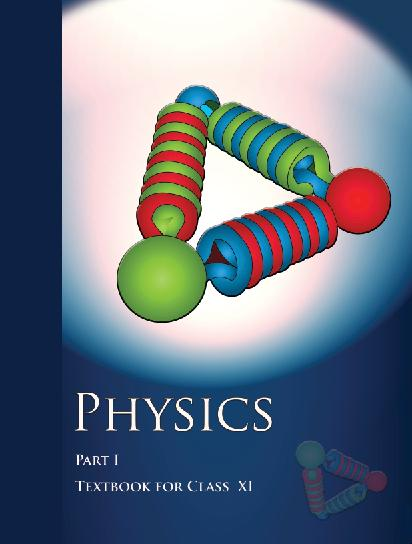## NCERT Class 11 Physics Chapter wise Solutions

• 1 – Physical World
• 2 – Units and Measurements
• 3 – Motion in a Straight line
• 4 – Motion in a Plane
• 5 – Laws of Motion
• 6 – Work, Energy and Power
• 7 – System of Particles and Rotational motion
• 8 – Gravitation
• 9 – Mechanical Properties of Solids
• 10 – Mechanical properties of fluids
• 11 – Thermal Properties of matter
• 12 – Thermodynamics
• 13 – Kinetic Theory
• 14 – Oscillations
• 15 – Waves

## CHAPTER 14 WAVES

• 15.1 Introduction
• 15.2 Transverse and longitudinal waves
• 15.3 Displacement relation in a progressive wave
• 15.4 The speed of a travelling wave
• 15.5 The principle of superposition of waves
• 15.6 Reflection of waves
• 15.7 Beats
• 15.8 Doppler effect

## NCERT Solutions For Class 11 Physics Waves

1. A string of mass 2.50 kg is under a tension of 200 N. The length of the stretched string is 20.0 m. If the transverse jerk is struck at one end of the string, how long does the disturbance take to reach the other end?

2. A stone dropped from the top of a tower of height 300 m high splashes into the water of a pond near the base of the tower. When is the splash heard at the top given that the speed of sound in air is 340 m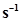? (g= 9.8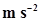)

3. A steel wire has a length of 12.0 m and a mass of 2.10 kg. What should be the tension in the wire so that speed of a transverse wave on the wire equals the speed of sound in dry air at 20 °C = 343 m?

4. Use the formula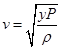to explain why the speed of sound in air

(a) is independent of pressure,

(b) increases with temperature,

(c) increases with humidity.

5. You have learnt that a travelling wave in one dimension is represented by a function y = f (x, t)where x and t must appear in the combination x–v t or x + v t, i.e. y = f (x ± v t). Is the converse true? Examine if the following functions for y can possibly represent a travelling wave:

(a)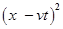(b)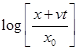(c)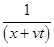6. A bat emits ultrasonic sound of frequency 1000 kHz in air. If the sound meets a water surface, what is the wavelength of

(a) the reflected sound,

(b) the transmitted sound? Speed of sound in air is 340 m s-1 and in water 1486 m.

7. A hospital uses an ultrasonic scanner to locate tumours in a tissue. What is the wavelength of sound in the tissue in which the speed of sound is 1.7 km? The operating frequency of the scanner is 4.2 MHz.

8. A transverse harmonic wave on a string is described by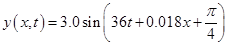……..(i)

Where x and y are in cm and t in s. The positive direction of x is from left to right.

(a) Is this a travelling wave or a stationary wave?

If it is travelling, what are the speed and direction of its propagation?

(b) What are its amplitude and frequency?

(c) What is the initial phase at the origin?

(d) What is the least distance between two successive crests in the wave?

9. For the wave described in Exercise 15.8, plot the displacement (y) versus (t) graphs for x = 0, 2 and 4 cm. What are the shapes of these graphs? In which aspects does the oscillatory motion in travelling wave differ from one point to another: amplitude, frequency or phase?

10. For the travelling harmonic wave

y (x, t) = 2.0 cos 2π (10t – 0.0080x + 0.35)

Where x and y are in cm and t in s. Calculate the phase difference between oscillatory motion of two points separated by a distance of

(a) 4 m,

(b) 0.5 m,

(c),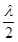(d)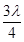11. The transverse displacement of a string (clamped at its both ends) is given by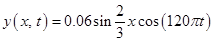Where x and y are in m and t in s. The length of the string is 1.5 m and its mass is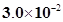kg.

(a) Does the function represent a travelling wave or a stationary wave?

(b) Interpret the wave as a superposition of two waves travelling in opposite directions. What is the wavelength, frequency, and speed of each wave?

(c) Determine the tension in the string.

12. (i) For the wave on a string described in Exercise 15.11, do all the points on the string oscillate with the same (a) frequency, (b) phase, (c) amplitude? Explain your answers. (ii) What is the amplitude of a point 0.375 m away from one end?

13. Given below are some functions of x and t to represent the displacement (transverse or longitudinal) of an elastic wave. State which of these represent (i) a traveling wave, (ii) a stationary wave or (iii) none at all:

(a) y = 2 cos (3x) sin (10t)

(b)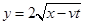(c) y = 3 sin (5x –0.5t) + 4 cos (5x–0.5t)

(d) y = cos x sin t + cos 2x sin 2t

14. A wire stretched between two rigid supports vibrates in its fundamental mode with a frequency of 45 Hz. The mass of the wire is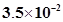kg and its linear mass density is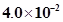kg m-1. What is (a) the speed of a transverse wave on the string, and (b) the tension in the string?

15. A metre-long tube open at one end, with a movable piston at the other end, shows resonance with a fixed frequency source (a tuning fork of frequency 340 Hz) when the tube length is 25.5 cm or 79.3 cm. Estimate the speed of sound in air at the temperature of the experiment. The edge effects may be neglected.

16. A steel rod 100 cm long is clamped at its middle. The fundamental frequency of longitudinal vibrations of the rod is given to be 2.53 kHz. What is the speed of sound in steel?

17. A pipe 20 cm long is closed at one end. Which harmonic mode of the pipe is resonantly excited by a 430 Hz source? Will the same source be in resonance with the pipe if both ends are open? (Speed of sound in air is 340 m).

18. Two sitar strings A and B playing the note ‘Ga‘ are slightly out of tune and produce beats of frequency 6 Hz. The tension in the string A is slightly reduced and the beat frequency is found to reduce to 3 Hz. If the original frequency of A is 324 Hz, what is the frequency of B?

19. Explain why (or how):

(a) In a sound wave, a displacement node is a pressure antinode and vice versa,

(b) Bats can ascertain distances, directions, nature, and sizes of the obstacles without any “eyes”,

(c) A violin note and sitar note may have the same frequency, yet we can distinguish between the two notes,

(d) Solids can support both longitudinal and transverse waves, but only longitudinal waves can propagate in gases, and

(e) The shape of a pulse gets distorted during propagation in a dispersive medium.

20. A train, standing at the outer signal of a railway station blows a whistle of frequency 400 Hz in still air.

(i) What is the frequency of the whistle for a platform observer when the train

(a) approaches the platform with a speed of 10 m,

(b) recedes from the platform with a speed of 10 m?

(ii) What is the speed of sound in each case? The speed of sound in still air can be taken as 340 m.

21. A train, standing in a station-yard, blows a whistle of frequency 400 Hz in still air. The wind starts blowing in the direction from the yard to the station with at a speed of 10 m. What are the frequency, wavelength, and speed of sound for an observer standing on the station’s platform? Is the situation exactly identical to the case when the air is still and the observer runs towards the yard at a speed of 10 m? The speed of sound in still air can be taken as 340 m.

22. A travelling harmonic wave on a string is described by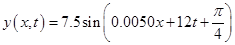(a) What are the displacement and velocity of oscillation of a point at x = 1 cm, and t = 1 s? Is this velocity equal to the velocity of wave propagation?

(b) Locate the points of the string which have the same transverse displacements and velocity as the x = 1 cm point at t = 2 s, 5 s and 11 s.

23. A narrow sound pulse (for example, a short pip by a whistle) is sent across a medium. (a) Does the pulse have a definite (i) frequency, (ii) wavelength, (iii) speed of propagation? (b) If the pulse rate is 1 after every 20 s, (that is the whistle is blown for a split of second after every 20 s), is the frequency of the note produced by the whistle equal to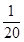or 0.05 Hz?

24. One end of a long string of linear mass density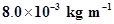is connected to an electrically driven tuning fork of frequency 256 Hz. The other end passes over a pulley and is tied to a pan containing a mass of 90 kg. The pulley end absorbs all the incoming energy so that reflected waves at this end have negligible amplitude. At t = 0, the left end (fork end) of the string x = 0 has zero transverse displacement (y = 0) and is moving along positive y-direction. The amplitude of the wave is 5.0 cm. Write down the transverse displacement y as function of x and t that describes the wave on the string.

25. A SONAR system fixed in a submarine operates at a frequency 40.0 kHz. An enemy submarine moves towards the SONAR with a speed of 360 km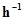. What is the frequency of sound reflected by the submarine? Take the speed of sound in water to be 1450 m.

26. Earthquakes generate sound waves inside the earth. Unlike a gas, the earth can experience both transverse (S) and longitudinal (P) sound waves. Typically the speed of S wave is about 4.0 km s-1, and that of P wave is 8.0 km. A seismograph records P and S waves from an earthquake. The first P wave arrives 4 min before the first S wave. Assuming the waves travel in straight line, at what distance does the earthquake occur?

27. A bat is flitting about in a cave, navigating via ultrasonic beeps. Assume that the sound emission frequency of the bat is 40 kHz. During one fast swoop directly toward a flat wall surface, the bat is moving at 0.03 times the speed of sound in air. What frequency does the bat hear reflected off the wall?

## NCERT Solutions for Class 11 Physics

NCERT Solutions Class 11 Physics PDF (Download) Free from myCBSEguide app and myCBSEguide website. Ncert solution class 11 physics includes text book solutions from both part 1 and part 2. NCERT Solutions for CBSE Class 11 Physics have total 15 chapters. 11 Physics NCERT Solutions in PDF for free Download on our website. Ncert physics class 11 solutions PDF and physics ncert class 11 PDF solutions with latest modifications and as per the latest CBSE syllabus are only available in myCBSEguide

## CBSE app for Class 11

To download NCERT Solutions for class 11 Physics, Chemistry, Biology, History, Political Science, Economics, Geography, Computer Science, Home Science, Accountancy, Business Studies and Home Science; do check myCBSEguide app or website. myCBSEguide provides sample papers with solution, test papers for chapter-wise practice, NCERT solutions, NCERT Exemplar solutions, quick revision notes for ready reference, CBSE guess papers and CBSE important question papers. Sample Paper all are made available through the best app for CBSE students and myCBSEguide websit## Test Generator

Create Papers with your Name & Logo